# Perturbed Magnetic Field

Let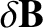be the perturbed magnetic field associated with a tearing mode to which the plasma is subject. Now,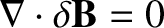, so we obtain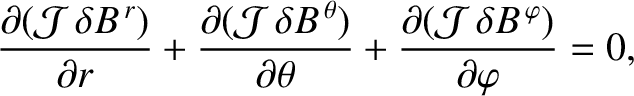(14.34)

where use has been made of Equation (14.13).

It is easily demonstrated from Equations (14.1)–(14.5) that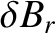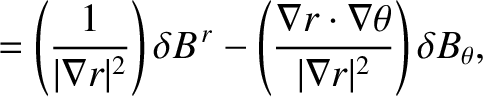(14.35)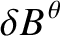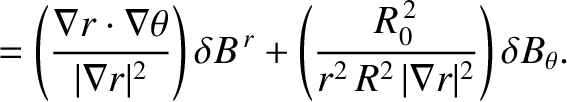(14.36)

Suppose, for the moment, that the tearing perturbation has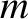periods in the poloidal direction and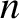periods in the toroidal direction. Let us adopt the simplifying approximation that the perturbed current density,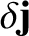, is negligible in the regions lying between the various rational surfaces in the plasma . Given that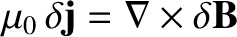, it follows from Equations (14.13)–(14.15) that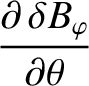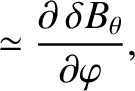(14.37)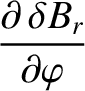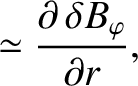(14.38)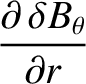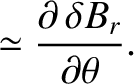(14.39)

Assuming that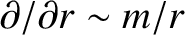, the previous three equations imply that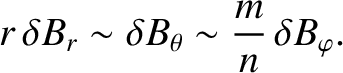(14.40)

Hence, we deduce that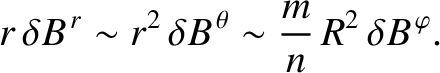(14.41)

Consequently, the final term on the left-hand side of Equation (14.34) is of order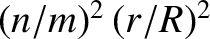smaller than the other two terms. Let us assume that this final term is negligible, as would be the case in a large aspect-ratio (i.e.,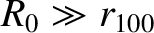) torus. It follows that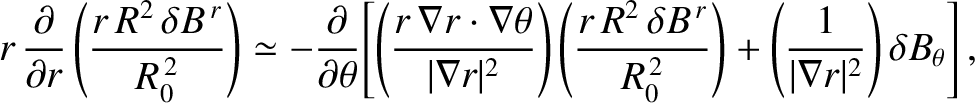(14.42)

where use has been made of Equation (14.3), (14.35), and (14.36). Finally, Equations (14.35) and (14.39) yield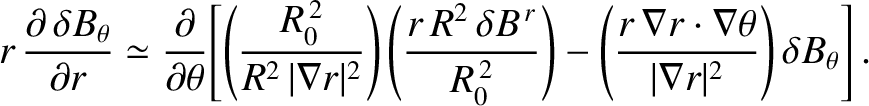(14.43)

Let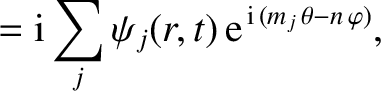(14.44)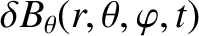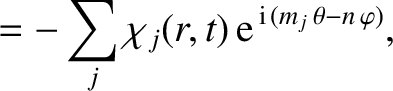(14.45)

where the sum is over all relevant poloidal harmonics of the perturbed magnetic field. Here, we are now taking account of the fact that a tearing mode in a toroidal tokamak plasma possesses a unique toroidal mode number, but consists of many coupled poloidal harmonics with different poloidal mode numbers [5,6,11,14,22,31]. Operating on Equations (14.42) and (14.43) with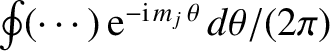, we obtain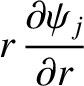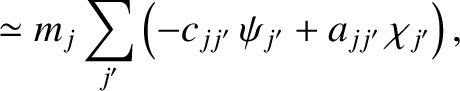(14.46)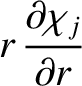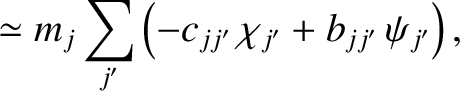(14.47)

where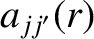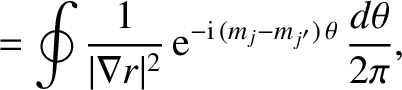(14.48)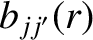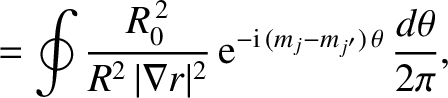(14.49)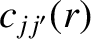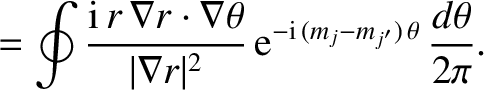(14.50)

Incidentally, we can recover the approximate relations (14.46) and (14.47) from the completely general analysis of Reference  by neglecting the equilibrium plasma current, as well as by taking the limit that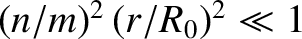. This procedure is roughly equivalent to neglecting the term involving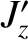in the cylindrical tearing mode equation, (3.60). Hence, by analogy with this equation, we would expect our toroidal tearing mode to be classically stable (given that the drive for the classical tearing instability in the cylindrical tearing mode equation derives from the term involving). However, this does not preclude the possibility that our toroidal tearing mode could be unstable as a neoclassical tearing mode. (See Chapter 12.)

Finally, it is readily demonstrated that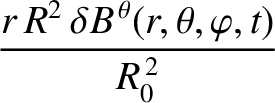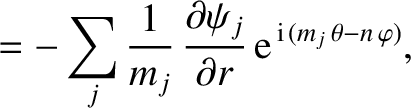(14.51)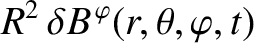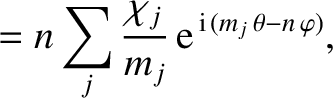(14.52)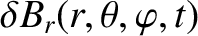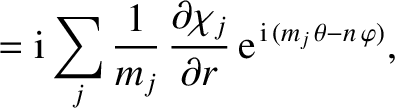(14.53)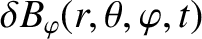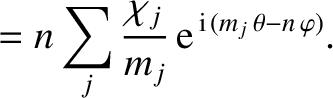(14.54)